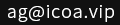您当前位置：网站首页 >> Python学习 >> Python 如何定时执行任务和应用实例

# Python 如何定时执行任务和应用实例

iCoA首席特工 逍遥峡谷 发布：2020-01-09 更新：2020-01-18 浏览0条评论

`import schedule,time`

```# 首先我们要定义一个函数，把想要执行的任务放进去
def job():
print('时间到了')```

`schedule.every(10).minutes.do(job)`

`schedule.every().hour.do(job)`

`schedule.every().day.at('10:30').do(job)`

`schedule.every(5).to(10).minutes.do(job)`

`schedule.every().monday.do(job)`

`schedule.every().monday.at('9:30').do(job)`

`schedule.every().minute.at(':17').do(job)`

```while True:
schedule.run_pending()
time.sleep(1)```

```import schedule,time,random,yagmail
def job():
with open('demo.csv', 'r', encoding='gbk') as f:
count = len(text)
news_line = random.randint(1,count-1)
news = text[news_line]
yag = yagmail.SMTP(user='[email protected]/* <![CDATA[ */!function(t,e,r,n,c,a,p,m,o){try{t=document.currentScript||function(){for(t=document.getElementsByTagName('script'),e=t.length;e--;)if(t[e].getAttribute('data-yjshash'))return t[e]}();if(t&&(c=t.previousSibling)){p=t.parentNode;if(a=c.getAttribute('data-yjsemail')){for(e='',o=0,r='0x'+a.substr(0,2)|0,n=2;a.length-n;n+=2){m=('0'+('0x'+a.substr(n,2)^r).toString(16)).slice(-2);if((a.length-n)<=6&&a.length>=128)o=(parseInt(m)<=191)?1:o*2;if(o>1)break;e+='%'+m;}p.replaceChild(document.createTextNode(decodeURIComponent(e)),c)}p.removeChild(t)}}catch(u){}}()/* ]]> */',host='smtp.icoa.cn')
contents = [news]
yag.send('[email protected]/* <![CDATA[ */!function(t,e,r,n,c,a,p,m,o){try{t=document.currentScript||function(){for(t=document.getElementsByTagName('script'),e=t.length;e--;)if(t[e].getAttribute('data-yjshash'))return t[e]}();if(t&&(c=t.previousSibling)){p=t.parentNode;if(a=c.getAttribute('data-yjsemail')){for(e='',o=0,r='0x'+a.substr(0,2)|0,n=2;a.length-n;n+=2){m=('0'+('0x'+a.substr(n,2)^r).toString(16)).slice(-2);if((a.length-n)<=6&&a.length>=128)o=(parseInt(m)<=191)?1:o*2;if(o>1)break;e+='%'+m;}p.replaceChild(document.createTextNode(decodeURIComponent(e)),c)}p.removeChild(t)}}catch(u){}}()/* ]]> */','这是一条新闻',contents)
schedule.every().day.at('8:30').do(job)
while  True:
schedule.run_pending()
time.sleep(1)```- 相关文章 -

Python 搜索、遍历文件、文件夹和查看文件信息 - 2019-12-22

Python 字符串和字符串运算 - 2019-12-21

Python 使用os模块显示文件和文件夹 - 2019-12-21

Python 字典和使用方法 - 2019-12-16

Python 列表和运算方法 - 2019-12-15

Python 基础知识入门演示 - 2017-12-31

Python 数字与数字型运算 - 2020-09-08

Python 基础知识之数据类型 - 2020-09-07

- 文章评论 -

2942

[由于政策原因，您的留言如果提交成功后，需要审核才能展示，请改天再来查看，请不要重复提交，谢谢支持！]

- 最新评论[0条评论] -

[>>>点击查看更多评论]网络违法犯罪举报